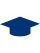polito.it# Time Between The Maximum And The Minimum Of One-dimensional Brownian MotionFrancesco Mori

Time Between The Maximum And The Minimum Of One-dimensional Brownian Motion.

Rel. Alessandro Pelizzola, Satya N. Majumdar, Paolo Biscari. Politecnico di Torino, Corso di laurea magistrale in Physics Of Complex Systems (Fisica Dei Sistemi Complessi), 2019

Abstract: The study of maxima and minima over a set of random variables is fundamental for the description of a plethora of phenomena, from climate studies to finance. Although the case of independent identically distributed random variables is well understood, in many relevant applications variables are strongly correlated and, without the assumption of independence, finding exact results becomes highly non trivial. An example of strongly correlated variables, for which it is possible to find exact solutions, are random walks. For instance, extrema of Brownian motion have been widely studied and analytical results are known, e.g. the probability distribution of the time $t_{max}$ at which a one-dimensional Brownian motion reaches its maximal value. However, nothing is known about probability distributions of quantities that depend on both the time of the maximum $t_{max}$ and the time of the minimum $t_{min}$. In this work, I present an exact solution for the probability density function $P(\Delta t=t_{min}-t_{max}|T)$ of the time $\Delta t$ between maximum and minimum of a one-dimensional Brownian motion starting from the origin and evolving up to time $T$. I show that the scaling form $P(\Delta t|T)=1/Tf_{BM}(\Delta t/T)$ holds and I compute analytically the function $f_{BM}$ using path-integral techniques. I extend this computation to a one-dimensional Brownian bridge, a Brownian motion with the additional constraint that the final position has to be zero. In this case, I find again a scaling form $P(\Delta t|T)=1/Tf_{BB}(\Delta t/T)$ with a different function $f_{BB}$. I use these results to compute, in both cases, the covariance between $t_{max}$ and $t_{min}$, showing that they are strongly anti-correlated. I also notice that $f_{BM}$ and $f_{BB}$ emerge in the description of extreme values of one-dimensional finite size fluctuating Kardar-Parisi-Zhang interfaces in the stationary state. Indeed, I study the probability distribution of the distance $\Delta \tau$ between the point at which the height of the interface is maximal and the point at which it is minimal. I show that the probability density function of $\Delta \tau$ has a scaling form with scaling function $f_{BM}$ and $f_{BB}$ for open boundary conditions and periodic boundary conditions, respectively. My results are confirmed by numerical simulations. Moreover, I show numerically that the scaling function $f_{BM}$ is also valid for discrete-time random walks in the limit of large number of steps if the distribution of the increments has tails decaying at least exponentially fast. Alessandro Pelizzola, Satya N. Majumdar, Paolo Biscari 2018/19 Electronic 52 Tesi secretata. Fulltext non presente Corso di laurea magistrale in Physics Of Complex Systems (Fisica Dei Sistemi Complessi) New organization > Master science > LM-44 - MATHEMATICAL MODELLING FOR ENGINEERING Université de Paris-Sud (Paris XI) (FRANCIA) CNRS LPTMS http://webthesis.biblio.polito.it/id/eprint/11722Modify record (reserved for operators)